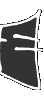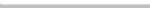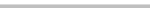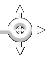m s e n e t * * * * m s e n e t * * * * m s e n e t * * * * m s e n e t * * * * m s e n e t * * * *b i o g r a p h y b i o g r a p h y b i o g r a p h y b i o g r a p h y b i o g r a p h yp o r t f o l i o p o r t f o l i o p o r t f o l i o p o r t f o l i o p o r t f o l i oc o m m e n t a r y c o m m e n t a r y c o m m e n t a r y c o m m e n t a r y c o m m e n t a r yh u m o r h u m o r h u m o r h u m o r h u m o rg o o d i e s g o o d i e s g o o d i e s g o o d i e s g o o d i e sall about this site, the latest installment of the venerable MSEnet, including why you have to scroll to the right to use it... everything you wanted to know but were rightly afraid to ask... a comprehensive display of my work to date, with examples of both web development and projects from my on-going education in architecture... a glimpse into how I think, demonstrated through academic essays and personal discourses... humorous tidbits that showcase my sense of humor... WARNING: you are likely to be offended... a small collection of random bits of code that do not fit into this site but are just too interesting to leave out...